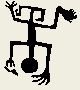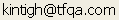Tools for Quantitative Archaeology    Email:KOETJE: Cluster Dominance Analysis

KOETJE performs a Monte Carlo analysis of the dominance of a specific class or type in a given cluster configuration; a modification of an idea suggested by Koetje (1987:44-47). The idea is to assess the degree to which perceived segregation of item classes, such as tool types, in different clusters might be due to chance. First, a measure of diversity, labeled K by Koetje, is calculated for each cluster and a weighted average of these K's, called K', is calculated for the complete configuration. The higher the individual cluster K, and configuration K' values, the more evenly distributed the types are within the clusters; the lower the average K' the greater the average dominance of types within clusters. Use of this program is described and illustrated in Howell and Kintigh (1966).

The significance of the K' value for the configuration is assessed through Monte Carlo runs in which types are randomly reassigned to points within the clusters with the constraint that in each run there is the same number of points of each type as there are in the original data. In the aggregate, a set of Monte Carlo runs provides a likelihood that a K' value as low or lower (as or more segregated) would be obtained, if there was no pattern to the assignment of types of points by cluster. (This need not be run on the results of a cluster analysis, it can be run on any cross-classification.)

The program works with the .PLT file produced by a k-means run in which a tabulation variable was specified. It can also be used on separate data sets consisting of counts of artifact classes by cluster as might be derived from some other quantitative or visual clustering procedure.

K is a close quantitative relative of Simpson's measure of ecological dominance or concentration, C (Pielou 1975: 8-9; 1977: 309-311). Assume that a set of N tools is divided into T types with the counts x1, x2...xT. By definition, the types appear in the proportions p1, p2,...pT, where Σpi=1. It turns out that the probability that any two artifacts chosen at random from the collection will be of the same type is C=Σpi². If a collection is dominated by a single type, this value will be high; if there is only one type, C=1. Conversely the value reaches a minimum if the objects are exactly evenly distributed among the types, Cmin=1/T. It turns out that K=(1-C)/(1-Cmin). Alternatively, K=(1-Σpi²)(1-1/T).

Because of the close relationship between K and C the Koetje program has been written to use Simpson’s C and to measure dominance rather than Koetje's measure of homogeneity, K (I find “homogeniety” confusing in this context anway). Use of either measure results in the same probabilities, which is to say the same substantive result, but of course the actual values of C and K will differ. There is no real advantage to the evenness measure K over the concentration measure C, and C is well established in the literature. (It also makes more sense to me to talk about concentration or dominance of certain classes in certain clusters, rather than homogeneity.) In any event, because of the these measures are essentially inverses of one another, high values of C are usually what one is looking for rather than low values of K. The probabilities have the same meaning.

Going back to the Koetje program, C is calculated for the type distribution within each cluster. C' summarizes these with an average weighted by the number of items in each cluster. Results of the Monte Carlo analysis are displayed in two forms. The mean and standard deviation of the randomly derived C' provide a sense of its distribution; the observed C' can then be described as a Z score based on the random mean and standard deviation. The percentile location of the observed C' within the random distribution of C' can be derived from the table by locating the original data C'. In the sample presented below, the original C' of 0.58 is at the 100th percentile. This indicates that none of the random runs got a value this high indicating that the concentration of types by cluster is most likely not due to chance.

The Koetje program also includes information (not suggested by Koetje) on the C values derived for the individual clusters in the random runs. Est.Prob, the probability of finding a C value as high or higher than the observed C by chance is estimated by the fraction of random runs with C values for the cluster as high or higher than the observed C. The mean and std of each cluster's C for the random runs are provided for each cluster along with the normal probability of the Z score deviation of the actual data from the random runs. However C and C' are not, in general, normally distributed so normal probabilities should not be considered accurate estimates. The minimum and maximum C within the set of random runs is also included. However with more random runs, the minimum will generally decrease and the maximum increase whereas the Est.Prob, Mean and Std should stabilize.

Ordinarily, one is interested in situations in which low probabilities are associated with C' and C, indicating that types are unevenly distributed, or concentrated within clusters. However, it should be noted that very high probabilities also indicate unlikely events. For example a C' with an estimated probability of 0.96 indicates that only 4% of the time will random allocations produce a set of type distribution as even as the observed one. A very high probability of C' would suggest that there are processes that are producing even (not random or type-dominated) allocations across the clusters. However, if C values for the clusters in any configuration vary widely, the effect is likely a mechanical one in which a strong concentration of a common type in one cluster (which should have a high C and low probability) may produce artificially high concentrations of relatively less common types (resulting in unusually even distributions) in other clusters (which will have a low C and high probability).

SEQUENCE OF PROGRAM PROMPTS

Read Cluster Assignments from K-means .PLT File {Y} ?

Should input be read from a k-means plot file or a separate Antana format file.

Read Cluster Assignments from k-means .PLT File {Y} ?

Indicate if kmeans output is to be analyzed.

K-means Plot File {.PLT} ?

Name of the input file produced by k-means.

Cluster Level to Analyze ?

From the .PLT file, select the cluster configuration to analyze.

Output File for Koetje Analysis Results {.TXT} ?

Location of output listing. Type PRN to send output directly to printer.

Use [C] or Unbiased [E]stimator of C {C} ?

Generally C is fine. The program will use Pielou’s unbiased estimator if you wish.

Random Generator Seed (0 to set from clock) {0} ? 0

File of Type Counts by Cluster {.ADF} ?

An antana file, in which the rows represent the clusters in a specific configuration and the columns the different types. The values in the file represent for each cluster in the configuration, the number of points of the type corresponding to that column.

Number of Random Trials {100} ?

Enter the number of trials to develop an expectation for the distributions of point types by cluster (up to 2000).

xxx Trials

This keeps you informed of the progress of the analysis, counting up the trials performed.

Perform Another Analysis {N} ?

The analysis can be repeated for another configuration.

Program End

PROGRAM INPUT

```8 5
12  0 0 1 83
8  5 0 6 60
9  0 0 8 59
1  0 7 2  7
8  0 0 0  0
9  1 0 2 48
2 31 0 5  5
3 45 50 4 9```

PROGRAM OUTPUT

```Koetje Homeogeneity Expectation  Random Number Seed: 744623413

Input Data - 8 Clusters
1:  12   0   0   1  83
2:   8   5   0   6  60
3:   9   0   0   8  59
4:   1   0   7   2   7
5:   8   0   0   0   0
6:   9   1   0   2  48
7:   2  31   0   5   5
8:   3  45  50   4   9

Monte Carlo Estimation for 5 Types & 8 Clusters - Simpson's C
Original Data C': 0.5864;  0.0% (0.000) of 2000 trials have C'>=Observed C'
Mean Random C'= 0.3712   Random Std C'= 0.0028

Original Data  |                  Random Trials
Clus  Size      C |Est.Prob Mean C  Std C  Min C  Max C
1    96  0.763 |   0.000  0.367  0.039  0.266  0.527
2    79  0.597 |   0.000  0.368  0.044  0.250  0.537
3    76  0.628 |   0.000  0.370  0.046  0.247  0.544
4    17  0.356 |   0.653  0.401  0.103  0.218  0.889
5     8  1.000 |   0.009  0.443  0.149  0.219  1.000
6    60  0.664 |   0.000  0.374  0.053  0.241  0.606
7    43  0.549 |   0.008  0.375  0.062  0.223  0.642
8   111  0.376 |   0.367  0.366  0.035  0.266  0.485

* C and C' are non-normal, probabilities approximate

Random C' Percentile Distribution (based on 2000 trials)
0%     5%    10%    15%    20%    25%    30%    35%    40%    45%    50%
0.3651 0.3673 0.3679 0.3685 0.3689 0.3693 0.3696 0.3699 0.3702 0.3705 0.3708
50%    55%    60%    65%    70%    75%    80%    85%    90%    95%   100%
0.3708 0.3712 0.3716 0.3720 0.3724 0.3729 0.3735 0.3742 0.3750 0.3763 0.3853```

 Home Top Overview Ordering Documentation

Page Last Updated - 02-Jun-2007